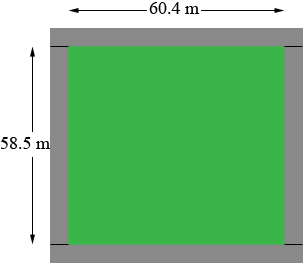SEARCH HOMEMath Central Quandaries & QueriesQuestion from Paige, a student: an area of the school courts is 58.5m x 60.4m, The spectator area is to be 2m wide and the concrete has to be 10cm deep Please can you calculate the volume of concrete needs for the spectator area?Paige,You can view the concrete to be added as 4 rectangular slabs. The two on the sides in my diagram are 58.5 m long, 2 m wide and 10 cm thick. What is the volume of each of these slabs? (You should convert the 10 cm thickness to meters so the volume is in cubic meters.) How long are the slabs at the top and bottom in my diagram? What are their volumes? Add the volumes of the 4 slab to find the total volume of the concrete needed.

PennyMath Central is supported by the University of Regina and The Pacific Institute for the Mathematical Sciences.